# A Genetic Algorithm for Three-Stage Hybrid Flow Shop Scheduling Problem with Dedicated Machines

A Genetic Algorithm for Three-Stage Hybrid Flow Shop Scheduling Problem with Dedicated Machines
Department of Industrial Engineering, National Engineering School of Tunis, University of Tunis El Manar, Tunis 1002, Tunisia

Corresponding Author Email:
asma.ouledbedhief@enit.utm.tn
Page:
357-368
|
DOI:
https://doi.org/10.18280/jesa.530306
26 May 2020
|
Accepted:
14 June 2020
|
Published:
31 July 2020
| Citation

OPEN ACCESS

Abstract:

In recent years, many studies have been carried out to solve scheduling problems with dedicated machines. But, few of them have considered the case of more than two stages. This paper aims at filling this gap by addressing the three-stage hybrid flow shop scheduling problem with two dedicated machines in stage 3. The objective is to minimize the maximum completion time (makespan). Since the genetic algorithm is very effective at finding optimal solutions to a wide variety of problems, this paper proposes an improved genetic algorithm (IGA). It uses more than one crossover operator and is based on a local search method (2-opt). The impact of different control parameters and operators on the outcomes of the basic genetic algorithm has been firstly tested by means of computational experiments, which can help us to undertake some observed shortages when developing our improved genetic algorithm. The results with different problem configurations demonstrate the effectiveness of IGA for solving the three-stage scheduling problem with dedicated machines, with a mean percentage deviation from the lower bound that did not exceed 0.5% and a very reasonable computational time. Besides, the simulation results show that our improved GA model outperforms an existing heuristic approach that has been previously proposed in the academic literature to deal with the same problem.

Keywords:

flow shop, dedicated machines, genetic algorithm, crossover, mutation, local search

1. Introduction

The hybrid flow shop (HFS) scheduling problem may arise in a large class of real manufacturing environments. It is a combination of the flow shop where all products flow successively from one stage to the next, and the parallel shop where several machines are present in at least one stage to complete the associate manufacturing phase. The HFS problem consists in assigning jobs to parallel machines and sequencing the jobs assigned to the same machine. Scheduling in such an environment with two or more stages is NP -hard, even when there is one machine in the first stage and two identical machines in the second stage .

In this paper, we consider a special type of HFS scheduling problem: the hybrid flow shop with dedicated machines, where a machine is said to be dedicated to a specific job type if this job can be processed only on the corresponding machine that is dedicated to that job.

In several industrial applications, dedicated machines consist to produce different types of a same basic product. For example, various types of products go through the same main operations of the production process, and then, they are processed on dedicated machines specific to each product type, such as in pharmaceutical industry, label sticker manufacturing , pottery production, furniture assembly , mass customization  and global manufacturing firms .

In these real manufacturing environments, the most of organizations with dedicated machines are composed of at least three stages of production. And, the HFS with dedicated machines with two or more stages is NP-hard in the strong sense [5, 6].

Several studies have considered this particular type of problem. But, the majority of these studies have focused on the two-stage configurations. The first study in the HFS problem with dedicated machines was done by Herrmann and Lee . They considered a two-stage HFS with one single machine in the first stage and two dedicated machines in the second stage and proved the NP-hardness of the problem for three objective functions: the makespan, the total completion time and the number of tardy jobs. Following their work, other studies considering two-stage configurations, are reported in the literature:

Some studies have proposed exact methods [7-9]. Riane et al.  developed a dynamic program in order to solve a two-stage hybrid flow shop problem with two dedicated machines in the second stage. Huang et al.  considered the same problem with constant setup times in the first stage. They examined the case where processing sequences of the two types of jobs are given and they proposed a dynamic programming algorithm. Hadda et al.  introduced a branch and bound method and presented a new dominance rule for this problem. Mosheiov et al.  proposed a linear integer program that minimizes the weighted number of tardy jobs in a two-stage HFS with m dedicated machines in the second stage.

For the case of several dedicated machines in stage 1, a set of dominance rules are presented and different polynomial cases are proposed by Yang et al. [11, 12].

Other studies have proposed heuristic approaches [13-15]. For the case of two dedicated machines in the first stage, Oguz et al.  considered the objective of minimizing the makespan and developed a heuristic approach based on the Johnson’s algorithm . Yang  investigated the same problem with respect to the total completion time minimization. The author proposed an optimal solution for the case where the processing times on the single machine of stage 2 are identical. Lin and Liao  studied the case of two-stage HFS with setup times and due dates in a label sticker manufacturing company. The aim was to minimize the weighted maximum tardiness of jobs. To solve the problem, the authors proposed a heuristic approach with combined rules.

For more than two dedicated machines in the second stage, many heuristic approaches are developed in the papers [14, 15].

For the case of more than two stages, Riane et al.  considered a three-stage HFS with two dedicated machines in the second stage and one single machine in stages 1 and 3. They developed a dynamic programming-based heuristic and a branch and bound based heuristic. Ouled Bedhief et al.  studied the case of three-stage HFS with dedicated machines in stage 3. The authors studied a set of particular cases and proposed a heuristic approach for the general problem that is denoted by IH-DP.

The literature review shows that the scheduling problem with dedicated machines with more than two stages is not widely studied. Thus, the lack of studies considering three stages represents a step that can be taken to obtain a more thorough view on the problem. Furthermore, the most of research on HFS with dedicated machines focus on the development of heuristics, and optimal solutions for particular cases of the problem. To the best of our knowledge, there is no report on metaheuristics for the three-stage HFS problem with dedicated machines.

In this paper, we investigate the problem of minimizing the makespan in a three-stage hybrid flow shop with dedicated machines. It can be defined as follows: We consider a set J={1,2…n} of n jobs to be executed in a three-stage HFS where there is one single machine in each of stages 1 and 2, denoted as Mj, j={1,2} and two dedicated machines Dk, k={1,2} in stage 3. Each job i must be processed consecutively on M1, and M2, with a processing time pij,j={1,2} and depending on its type, it will be further processed on a dedicated machine Dk, with a processing time dpik, k={1,2}. The objective is to find a feasible schedule $\pi=\left(\pi_{1}, \pi_{2}, \ldots, \pi_{n}\right)$ that minimizes the makespan (the maximum completion time) denoted by Cmax. For this problem, the set of permutation schedules is dominant . Following the notation of $\alpha|\beta| \gamma$ proposed by Graham et al. , we denote this problem as 3FHD|1,1,2|Cmax.

This problem appears from a real-life application that can be found in a liquid detergent industry. Figure 1 shows the schematic of its production process: The shop produces two types of products, which are sold in a local market or an export market. The products undergo three different operations: blow molding, filling and labeling.

The workshop is composed of a unique blow molding machine. Then, the resulting bottles are transferred to the liquid filling machine in stage 2. The last operation consists on labeling. There are two dedicated machines able to label bottles sold in local and export markets (Figure 1).

Due to the NP-hardness of the 3FHD|1,1,2|Cmax problem, it is more difficult to solve it using exact methods when the number of jobs increases. Thus, we need to develop efficient heuristics or metaheuristics to find good approximate solutions in a very reasonable computational time. In this paper, we propose a genetic algorithm, since this later is successfully applied for scheduling problems.

The rest of the paper is organized as follows:

Section 2 describes the implementation details of our basic genetic algorithm. Section 3 presents a set of computational experiments employed for evaluating the effects of control parameters and operators on the outcomes of this genetic algorithm. Based on these experiments, we propose in Section 4, an improved genetic algorithm (IGA) to solve the problem. We further evaluate its performance to minimize the makespan. Finally, Section 5 concludes the paper.

## 1.jpg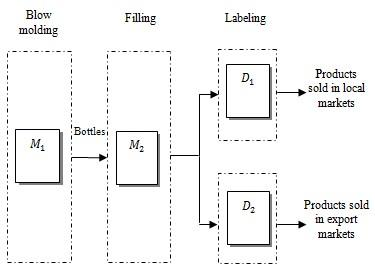Figure 1. Schematic of the production process in a liquid detergent industry

2. Basic Genetic Algorithm

The genetic algorithm was originally proposed by Holland  and since, it was usually applied to solve many combinatorial optimization problems. This metaheuristic uses a set of solutions called a population that can be changed using different operators such as selection, crossover, mutation and insertion, to provide a high-quality solution.

A solution is referred to as an individual or a chromosome. Each chromosome is composed of many genes, and a gene can also be identified as an allele. The process of a genetic algorithm begins with an initial population of a fixed number (population size) of individuals. Each individual of the population is evaluated by its fitness value which is related to the objective function of the problem. Then, using a selection method, the most fitted individuals in the population are selected as parents to create new individuals called offspring, according to crossover and mutation operators. These offspring are, next, reinserted into the current population based on their fitness and by keeping the population size. Such a process is reiterated until a stopping criterion is reached.

In this paper, we use this general structure to construct our genetic algorithm. In the following, we present, in detail, the implementation of our basic genetic algorithm proposed for the 3FHD|1,1,2|Cmax problem.

2.1 Solution encoding

Many encoding approaches exist in the literature for solving scheduling problems. We distinguish between a direct and indirect representation. In this work, we consider a direct encoding representation, where a solution is directly encoded into the chromosome. Thus, a chromosome is a sequence (a permutation) of n jobs (1, 2 … n) corresponding to the job order in the three stages (See Figure 2).

## 2.jpg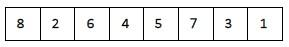Figure 2. A direct encoding representation

2.2 Initial population

The performance of a genetic algorithm depends on the initial population, which can be generated with different methods. In our genetic algorithm, the initial population is produced randomly to generate $P_{\text {size}}$  individuals, where the population size, $P_{\text {size}}$, is one of the control parameters to be kept constant through the generations. In this work, we consider two population size values: 50 and 100, representing respectively a small population and a large population.

2.3 Fitness

The fitness value is related to the objective function of an individual. In this work, the fitness of an individual is defined by the makespan value ($C_{\max }$) of the corresponding schedule.

2.4 Selection

In the selection step, a set of individuals are selected from the current population to produce the offspring. Many selection methods are used in the literature for scheduling problems. In this study, we will focus on random, roulette wheel and tournament selection.

- Random selection: It consists in selecting randomly the individuals (as parents) from the current population.

- Roulette wheel selection: In this method, a roulette wheel with slots is used. Each slot is assigned to an individual of the population and sized proportional to its fitness value. Figure 3 describes in detail the different steps of the roulette wheel selection.

## 3.jpg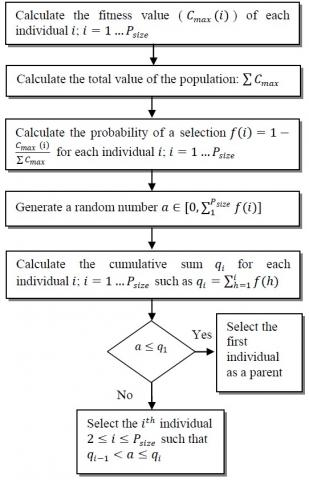Figure 3. Steps of the roulette wheel selection

## 4.jpgFigure 4. Steps of the tournament selection

-Tournament selection: This method has been introduced by Goldberg . We select randomly k individuals from the current population and we select the best one to become a parent (Figure 4).

In this study, we select $\frac{P_{\text {size}}}{2}$ individuals from the current population using one of these selection methods.

The selected individuals form a population that we denote as Pop$_{\text {select}}$. Once the individuals are selected, the crossover and mutation operators are used to generate new offspring.

2.5 Crossover

Typically, the crossover is the main genetic operator on which the performance of a genetic algorithm is very dependent. The crossover operator recombines two selected individuals (parents) called Parent1 and Parent2 to produce two new individuals (offspring) called Child1 and Child2, according to a crossover probability Pcross.

Several crossover operators have been proposed in the literature for scheduling problems. In this study, we choose four crossover operators, namely the One-Point Crossover (1X), Two-Point Crossover (2X), Order Crossover (OX) and Linear Order Crossover (LOX).

Furthermore, we introduce a new crossover operator which is denoted by NOX (New Order Crossover).

- One-point Crossover (1X):

Given two parent chromosomes, a random cut point is selected partitioning them into left and right portions. The left section of Parent1 (Parent2) is transferred to Child1 (Child2), and its right section is completed with the missing jobs in the same order that they appear in Parent2 (Parent1). (See Figure 5)

- Two-point Crossover (2X):

Given two parent chromosomes, two random cut points are selected partitioning them into a left, middle and right portions. The left and right sections of Parent1 (Parent2) are copied in Child1 (Child2), and its middle section is completed with the missing jobs in the same order that they appear in Parent2 (Parent1). (See Figure 6)

## 5.jpg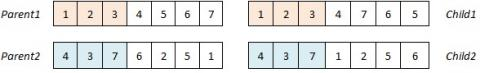Figure 5. Crossover operator (1X)

## 6.jpg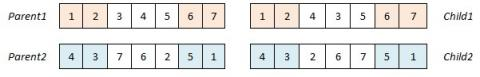Figure 6. Crossover operator (2X)

## 7.jpgFigure 7. Crossover operator (OX)

## 8.jpg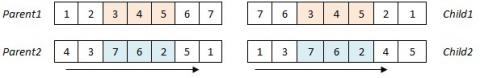Figure 8. Crossover operator (LOX)

## 9.jpgFigure 9. Crossover operator (NOX)

- Order Crossover (OX):

Given two parent chromosomes, two random cut points are selected partitioning them into left, middle and right portions. The middle section is copied from Parent1 (Parent2) into Child1 (Child2). The left and right sections are completed with the remaining jobs in the relative order of Parent2 (Parent1) starting after the second cut point. (See Figure 7)

- Linear Order Crossover (LOX):

Its procedure is similar to that of OX, with a slight modification: Once the substring between the two cut points is transferred from Parent1 (Parent2) to Child1 (Child2), this new job sequence genes is completed with the remaining jobs in the relative order of Parent2 (Parent1) starting from left to right. (See Figure 8)

- New Order Crossover (NOX):

The proposed crossover operator NOX combines the ideas of both OX and LOX.

It determines randomly two cut points, and for generating an offspring, the substring between these two points is transferred from Parent1 (Parent2) to Child1 (Child2). Then, starting after the second cut point of Child1 (Child2), (as in OX), the empty genes are filled with the missing jobs in the relative order of Parent2 (Parent1) scanning from left to right (as in LOX). (See Figure 9)

In this study, we consider two crossover probability values Pcross: 0.7 and 0.9, since similar values are usually used in genetic algorithms for HFS scheduling problems [23, 24]. All generated offspring form a population that we denote as POPchild.

2.6 Mutation

The use of a mutation helps the genetic algorithm to escape from a local minimum and prevents its premature convergence. The mutation is commonly used as a simple search operator which introduces a random gene or a chromosome change, according to a mutation probability $P_{\operatorname{mut}}$.

In this study, we consider two mutation operators: insertion and swap that are widely used in the literature for HFS scheduling problems .

- Insertion-mutation: This operator consists in selecting randomly a gene from the individual and inserting it in a random position. (See Figure 10)

## 10.jpg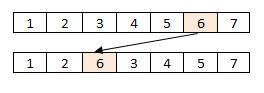Figure 10. Mutation operator (Insertion)

- Swap-mutation: This operator consists in selecting randomly two genes from the individual and exchanging their relative positions. (See Figure 11)

## 11.jpgFigure 11. Mutation operator (Swap)

The mutation probability $P_{\operatorname{mut}}$ is frequently small and often is mentioned ( $0.01 \leq P_{m u t} \leq 0.1$ ). Hence, we tested, in this study, Pmut=0.05 and 0.1.

2.7 Reinsertion method

In many proposed genetic algorithms, the new populations can include both parents and generated offspring and the population size is kept constant through the generations. In this study, offspring are inserted into the current population based on their fitness value. All individuals (parents and offspring) should be ranged in increasing order of their fitness value and the best $P_{\text {size}}$ individuals are considered as the new population of the next generation.

2.8 Stopping criterion

Several stopping criteria are considered in the literature such as maximum time limit, maximum number of iterations (generations), and no improvement in the best objective function value.

In this study, our genetic algorithm stops once the iteration number reaches the maximum number Iter$_{\max }$, chosen as 200 and 800.

The control parameters and operators of our basic genetic algorithm and their modalities that will be used later are summarized in Table 1.

The steps of our basic genetic algorithm can be described in the Figure 12 bellow.

Table 1. The parameters and operators used

 Parameters Population size: $P_{\text {Size}}$ 50; 100 Crossover probability: $P_{\text {cross}}$ 0.7; 0.9 Mutation probability: $P_{\text {must}}$ 0.05; 0.1 Maximum number of iterations: $Iter_{max}$ 200; 800 Operators Selection random, roulette wheel, tournament Crossover 1X; 2X; LOX; OX; NOX Mutation insertion; swap

## 12.jpg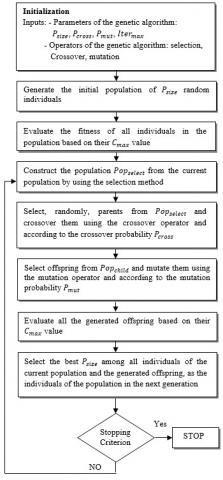Figure 12. Steps of the basic genetic algorithm

3. Computational Experiments

In order to provide an efficient version of the genetic algorithm to the 3FHD|1,1,2|Cmax problem, we conduct many experiments.

On one hand, we evaluate, separately, the effects of five crossover operators and two mutation operators on the outcomes of the basic genetic algorithm. We compare, for each selection method, the crossover operators without the mutation step, and we compare the mutation operators without the crossover step. In this case, the population size and the maximum number of iterations are fixed respectively at 100 and 800.

On the other hand, we study the interactive effects of the crossover and mutation operators on the performance of the genetic algorithm. We test several combinations of crossover and mutation operators under different problem sizes. Each combination is a version of the genetic algorithm. In this case, a preliminary computational test with factorial experiments design is performed to set the best values of the control parameters for each version of the genetic algorithm.

Our genetic algorithm is implemented using C++ and obtained in a personal computer with an Intel 2.50 GHz CPU and 1.96 GB RAM.

The results are presented in terms of mean percentage deviation MPD of the makespan from the lower bound.

The MPD of an algorithm is defined as:

$M P D=\sum_{i=1}^{20} \frac{P D(i)}{20}$  (1)

where, PD(i) is the percentage deviation of instance i such as:

$P D(i)=\frac{\left[C_{\max }(i)-L B(i)\right]}{L B(i)} * 100$  (2)

We note that $C_{\max }(i)$ represents the makespan value obtained by the genetic algorithm for a problem instance i and LB(i) denotes the lower bound value of instance i, which is calculated as presented in the paper .

3.1 Data generation

We carried out the computational experiments on five classes of test problems which are generated randomly. In each class, first and second-stage processing times are random integers from a uniform distribution from 1 to 20, denoted by $p_{i 1} \sim U[1,20]$ and $p_{i 2} \sim U[1,20]$. The ease of finding a satisfying solution for the studied problem depends on whether there is a balance between average workloads of dedicated machines and the total workload on the single machines. Hence, the processing times of jobs on the dedicated machines are generated randomly from the following uniform distributions :

CLASS 1: $d p_{i k} \sim U[1,20] \forall k \in\{1,2\}$

CLASS 2: $d p_{i k} \sim U[1,60] \forall k \in\{1,2\}$

CLASS 3: $d p_{i k} \sim U[20,40] \forall k \in\{1,2\}$

CLASS 4: $d p_{i k}=p_{i 2}+5$ with $k \in\{1,2\}$

CLASS 5: $d p_{i k}=p_{i 2}+10$ with $\forall k \in\{1,2\}$

Moreover, each job has an integer parameter which defines its processing on the dedicated machine in stage 3. This parameter is chosen randomly between D1 and D2, since there is no preference between the two dedicated machines. Several instances of various sizes are generated (N=40,80120,160,200) for each of the five classes.

3.2 A comparative study of crossover operators

In this paragraph, we compare the performance of five crossover operators to minimize the makespan, without the mutation step.

3.2.1 Computational results

To conduct experiments, we set the crossover probability $P_{\text {cross}}$ to 0.9 and we set the mutation probability $P_{m u st}$  to 0. We recall that the crossover operators used in this study are 1X, 2X, OX, LOX and NOX.

The comparative results are shown in Figures 13-17 below.

We note that this comparison is carried out for each problem size and selection method, where we generate 20 instances for each pair of operators (selection, crossover) and a problem size. For each instance, we evaluate the percentage deviation of the makespan from the lower bound, and the results are presented in terms of mean percentage deviation MPD for each class of test problems. Since the results are similar for all problem sizes, we present the comparative results of crossover operators for only a problem size equal to 120 jobs (Figures 13-17).

## 13.jpgFigure 13. Comparison of crossover operators for CLASS 1 problems

## 14.jpgFigure 14. Comparison of crossover operators for CLASS 2 problems

## 15.jpgFigure 15. Comparison of crossover operators for CLASS 3 problems

## 16.jpgFigure 16. Comparison of crossover operators for CLASS 4 problems

## 17.jpgFigure 17. Comparison of crossover operators for CLASS 5 problems

3.2.2 Discussion

The results show, firstly, that 2X is worse than all other crossover operators for solving the 3FHD|1,1,2|Cmax problem, where it gives the largest mean percentage deviation of the Cmax value from the lower bound. In fact, the makespan calculation, in our case study, is mainly conditioned by the choice of jobs to be placed at the beginning of the sequence and the idle-time that can be produced. And, it is also conditioned by the choice of jobs to be placed at the end of the sequence and the time that can be taken to finish the last operations. However, by preserving the beginning and the end of the sequence, the modification proposed by 2X is not relevant and often does not make an improvement in the makespan.

Furthermore, we can observe that for all cases of test problems, the roulette wheel selection outperforms tournament and random selection methods achieving best solution quality. Indeed, in the roulette wheel selection, individuals with higher fitness have more probability of selection, but it can be also possible to select low fit individuals, which may ensure certain heterogeneity and avoid the premature convergence of our algorithm as problem size increases.

According to these results, we limit our choice, in the genetic algorithm, to the roulette wheel as the selection method and we exclude the 2X crossover operator.

On the other hand, it is observed that the respective performances of the crossover operators (1X, OX, LOX and NOX) depend on the type of data. We find that the best crossover operator for CLASS 1 is 1X with a mean percentage deviation equal to 0.37%. For all cases of CLASS 3, CLASS 4 and CLASS 5, the proposed operator NOX outperforms the other crossover operators in finding near optimal solution, where the MPD values do not exceed 0.67%. While for CLASS 2, we find that LOX performs the best with a mean percentage deviation equal to 0.47%.

3.3 A comparative study of mutation operators

In this paragraph, we compare the performance of two mutation operators to minimize the makespan, without the crossover step.

3.3.1 Computational results

To conduct experiments, we set the mutation probability Pmut to 0.9 and we set the crossover probability Pcross to 0.

We recall that the mutation operators used in this study are insertion and swap.

The comparative results are shown in Figures 18-22 below.

## 18.jpgFigure 18. Comparison of mutation operators for CLASS 1 problems

## 19.jpgFigure 19. Comparison of mutation operators for CLASS 2 problems

## 20.jpg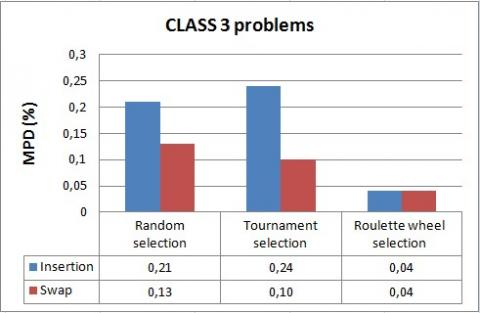Figure 20. Comparison of mutation operators for CLASS 3 problems

## 21.jpgFigure 21. Comparison of mutation operators for CLASS 4 problems

## 22.jpg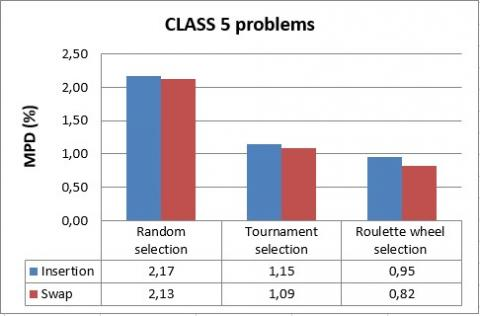Figure 22. Comparison of mutation operators for CLASS 5 problems

As well, the comparison is carried out for each problem size and selection method. We generate 20 instances for each pair of operators (selection, mutation) and a problem size. For each instance, we evaluate the percentage deviation of the makespan from the lower bound and the results are presented in terms of mean percentage deviation MPD for each class problems. Since the results are similar for all problem sizes, we present the comparative results of mutation operators for a problem size equal to 160 jobs (as shown in Figures 18-22 above).

3.3.2 Discussion

The simulation results show again that the roulette wheel selection outperforms tournament and random selection methods in terms of mean percentage deviation of the solution from the lower bound. Indeed, MPD values do not exceed 0.95% for the roulette wheel selection while, it can reach 2.13% for tournament and random methods.

Hence, we conclude that the roulette wheel selection method is the most suitable for solving the 3FHD|1,1,2|Cmax problem.

We can further observe that both of swap and insertion are effective to solve the problem, but in almost all cases, swap results are slightly better.

3.4 The interactive effects of crossover and mutation operators

In this section, we evaluate the interactive effects of crossover and mutation operators on the performance of the basic genetic algorithm. The objective is to determine the most appropriate combination of crossover and mutation operators for solving the 3FHD|1,1,2|Cmax problem. In this computational study, we use only NOX, LOX and 1X as crossover operators since each of them takes a first place for solving a given class problems, and we use insertion and swap as mutation operators. Also, we use the roulette wheel selection method since it gives the best results for all class problems. This means that we will run six versions of the proposed genetic algorithm. We will refer to these algorithms as 1X/insertion; 1X/swap; NOX/insertion; NOX /swap; LOX /insertion; and LOX /swap according to the genetic operators used in each of them.

3.4.1 Preliminary results

The performance of the six versions of the genetic algorithm depends on the determination of the control parameters used, which are the population size, crossover probability, mutation probability and maximum number of iterations (generations). In this paragraph, we seek to determine the suitable values of these control parameters for each version of the genetic algorithm.

Thus, before describing the computational results obtained for these genetic algorithms, we analyze their parameter setting. In this study, we use a factorial design of experiments, which has been very beneficial to design several metaheuristic approaches for solving scheduling problems. It consists to investigate the effects of different control parameters (input) on the results (output) and to choose the optimal values of these parameters. The factorial design of experiments that we use involves four parameters (factors), each having two possible values, such as Psize=50 and 100, Pcross=0.7 and 0.9, Pmut=0.05 and 0.1 and Itermax=200 and 800. The Combination of these parameters results in 2Χ2Χ2Χ2Χ2=16 runs for each genetic algorithm. In order to determine the best combination of parameters for each version of the proposed genetic algorithm, we consider CLASS 5. For each of the 16 runs, we generate randomly 10 instances of CLASS 5 with n=80. Hence, a total number of 16Χ10Χ6=960 runs are made in our preliminary computational experiments. Table 2 presents the parameter setting of each version of the genetic algorithm. The results are presented in terms of mean percentage deviation of the solution from the lower bound.

As it is seen in Table 2, the best parameter combination that obtains the smallest MPD is selected for each version of the genetic algorithm. Indeed, we find that the best combination for 1X/swap, NOX /swap and LOX /swap is Psize=100, Pcross=0.9, Pmut=0.1 and Itermax=800. For 1X/insertion and NOX/insertion, the best parameter combination is Psize=100, Pcross=0.7, Pmut=0.1 and Itermax=800. And, for LOX/ insertion, the best parameter combination is Psize=100, Pcross=0.7, Pmut=0.1 and Itermax=800. These parameter combinations will be used in the following computational experiments.

3.4.2 Computational results

This computational study aims to test the effects of different combinations of crossover and mutations operators on the performance of the basic genetic algorithm under different types of data. And thus, it is to determine the most appropriate combination of these genetic operators for solving the 3FHD|1,1,2|Cmax problem. The comparison of these combinations (versions of the genetic algorithm) is presented in Tables 3-7. The last column presents the average of CPU time of the six genetic algorithms.

Table 2. The computational results for parameter setting for each version of the basic genetic algorithm

 Parameter Combination $\left(P_{\text {size}} ; P_{\text {cross}} ; P_{\text {mut}} ;\right.$Iter $\left._{\text {max}}\right)$ Mean Percentage Deviation MPD (%) NOX/ swap NOX/ insertion LOX/ swap LOX/ insertion 1X/ swap 1X/ Insertion 50;0.7; 0.05; 200 1,28 1,45 0,96 1,08 1,16 1,12 50; 0.7; 0.05; 800 1,16 0,88 0,72 0,70 0,77 0,76 50; 0.7; 0.1; 200 1,20 1,23 0,99 1,21 1,28 1,08 50; 0.7; 0.1; 800 0,89 0,95 0,72 0,66 0,69 0,75 50; 0.9; 0.05; 200 1,25 1,33 1,04 0,82 1,40 1,10 50; 0.9; 0.05; 800 0,92 1,04 0,64 0,58 0,99 0,91 50; 0.9; 0.1; 200 1,32 1,25 1,04 1,02 1,27 1,26 50; 0.9; 0.1; 800 0,92 0,97 0,64 0,74 0,79 0,89 100; 0.7; 0.05; 200 1,19 1,26 0,99 0,89 1,14 1,27 100; 0.7: 0.05; 800 0,83 0,91 0,77 0,64 0,92 0,95 100; 0.7; 0.1; 200 1,17 1,16 1,02 0,86 0,93 0,99 100; 0.7; 0.1; 800 0,84 0,77 0,72 0,59 0,48 0,61 100; 0.9; 0.05; 200 1,22 1,29 0,86 0,88 0,90 1,20 100; 0.9; 0.05; 800 1,00 0,93 0,72 0,53 0,48 0,97 100; 0.9; 0.1; 200 1,08 1,21 0,88 1,07 0,92 1,07 100; 0.9; 0.1; 800 0,83 0,87 0,63 0,69 0,46 0,61

Table 3. Computational results of six genetic algorithms for CLASS 1 problems

 CLASS 1 MPD (%) Average CPU time of GAs(s) 1X/insertion LOX/insertion NOX/insertion 1X/swap LOX/swap NOX/ swap n=40 0.08 0.09 0.12 0.09 0.09 0.07 220.3 n=80 0.01 0.03 0.01 0.01 0.01 0.00 74.24 n=120 0.02 0.03 0.02 0.02 0.01 0.01 98.54 n=160 0.01 0.04 0.03 0.01 0.04 0.03 130.9 n=200 0.03 0.05 0.04 0.02 0.04 0.02 156.1

Table 4. Computational results of six genetic algorithms for CLASS 2 problems

 CLASS 2 MPD (%) Average CPU time of GAs(s) 1X/Insertion LOX/Insertion NOX/Insertion 1X/swap LOX/swap NOX/swap n=40 0.07 0.06 0.07 0.06 0.05 0.02 123.4 n=80 0.06 0.04 0.02 0.02 0.02 0.02 87.23 n=120 0.04 0.01 0.01 0.03 0.01 0.01 60.48 n=160 0.03 0.004 0.004 0.004 0.004 0.004 66.31 n=200 0.07 0.01 0.01 0.04 0.01 0.01 89.08

Table 5. Computational results of six genetic algorithms for CLASS 3 problems

 CLASS 3 MPD (%) Average CPU time of GAs(s) 1X/Insertion LOX/Insertion NOX/Insertion 1X/swap LOX/swap NOX/swap n=40 0.00 0.00 0.00 0.00 0.00 0.00 58.03 n=80 0.04 0.004 0.00 0.004 0.00 0.00 77.50 n=120 0.04 0.00 0.00 0.01 0.00 0.00 82.12 n=160 0.04 0.01 0.01 0.04 0.01 0.01 85.34 n=200 0.06 0.01 0.01 0.03 0.007 0.005 96.00

Table 6. Computational results of six genetic algorithms for CLASS 4 problems

 CLASS 4 MPD (%) Average CPU time of GAs(s) 1X/ Insertion LOX/ Insertion NOX/ Insertion 1X/swap LOX/swap NOX/swap n=40 0.68 0.77 0.81 0.68 0.58 0.81 2346 n=80 0.30 0.32 0.44 0.29 0.32 0.36 2422 n=120 0.15 0.18 0.22 0.15 0.17 0.18 2615 n=160 0.18 0.16 0.19 0.16 0.13 0.19 1546 n=200 0.05 0.07 0.08 0.05 0.07 0.08 723

Table 7. Computational results of six genetic algorithms for CLASS 5 problems

 CLASS 5 MPD (%) Average CPU time of GAs(s) 1X/insertion LOX/insertion NOX/insertion 1X/ swap LOX/swap NOX/swap n=40 1.62 1.73 1.82 1.25 1.45 1.67 2400 n=80 0.61 0.69 0.87 0.57 0.64 0.85 2612 n=120 0.38 0.21 0.45 0.25 0.21 0.50 2753 n=160 0.37 0.30 0.46 0.31 0.27 0.46 3020 n=200 0.31 0.20 0.27 0.25 0.20 0.27 3554
3.4.3 Discussion

The computational results show that the combination of crossover and mutation operators has a significant impact on the outcomes of the basic genetic algorithm, as the later performs better than a genetic algorithm running with only a crossover or a mutation operator. Also, for each crossover operator, it can be observed that the genetic algorithm which uses swap as a mutation operator performs better than the genetic algorithm which uses insertion, in terms of the mean percentage deviation (MPD).

Comparing the results of the six genetic algorithms, we notice that all of them are effective to solve the problem, as shown in Tables 3-7, where MPD values do not exceed 1.82%. However, we observe that for all problem cases of CLASS 1, CLASS 2 and CLASS 3, NOX/swap outperforms all other combinations of crossover and mutation operators, with a mean percentage deviation that does not exceed 0.07%. For CLASS 4 and CLASS 5, the best results are being shared between 1X/swap and LOX/swap with a mean percentage deviation that does not exceed respectively 1.25% and 1.45%.

Considering the results per problem class, we can see from Tables 3, 4 and 5, that for all instances of CLASS 1 and CLASS 2, MPD values of all genetic algorithms are very close to zero and do not exceed 0.12% while in almost all cases of CLASS 3, the MPD values are zero. This means that CLASS 1, CLASS 2 and CLASS 3 are relatively easy to solve by this genetic algorithm. However, CLASS 4 and CLASS 5, whose results are presented respectively in Tables 6 and 7, are more difficult as MPD values are the largest reaching 1.67%. Nevertheless, for most cases of such classes, MPD values of the genetic algorithms are slightly smaller in CLASS 4 than those in CLASS 5.

Tables 3-7 also present the average CPU times for solving the 3FHD|1,1,2|Cmax problem. For CLASS 1, CLASS 2 and CLASS 3, the CPU times are smaller than those of CLASS 4 and CLASS 5, since all genetic algorithms stop running once the best solution reaches the lower bound or once the iteration number reaches the maximum number. In general, the CPU times of the six different genetic algorithms are similar: As the number of jobs n  increases, the average CPU time increases for almost cases. This is an expect result due to the increasing search space.

These computational experiments allow us to set the control parameters of the basic genetic algorithm and to choose its different operators for each class problems. These parameters and operators can be summarized as follows:

• Controls parameters:
• Population size:100
• Crossover probability:0.9
• Mutation probability:0.1
• Maximum number of iterations:800
• Operators:
• Selection method: Roulette wheel selection
• Crossover operator: 1X, NOX and LOX
• Mutation operator: swap

In order to determine the crossover operator to use in our genetic algorithm, we examined the behavior of each of 1X, LOX and NOX by studying the evolution of the percentage deviation of the best solution (individual) within 800 iterations (generations). The Figures 23, 24 and 25 present, respectively, the evolution of the best solution obtained with LOX/swap, 1X/swap and NOX/swap within 800 iterations for a given instance.

## 23.jpgFigure 23. Evolution of the best individual within 800 generations for LOX/swap

## 24.jpgFigure 24. Evolution of the best individual within 800 generations for 1X/swap

## 25.jpgFigure 25. Evolution of the best individual within 800 generations for NOX/swap

The results show that the best solution, most often, is going to stagnate for several iterations, after which it changes. The stagnation of the makespan may be justified by the repetitive application of the same crossover operator which may allow the genetic algorithm stagnates and converges, in most cases, to a local minimum.

With the aim to obtain a better solution in a reasonable computational, we propose an improved genetic algorithm that we denote by IGA. It uses more than one crossover operator and a local search method (2-opt).

Next, we present in details our improved genetic algorithm for solving the 3FHD|1,1,2|Cmax problem.

4. Improved Genetic Algorithm (IGA)

In this section, we propose an improved genetic algorithm (IGA) to solve the problem. It is a solution to escape the stagnation of the makespan and provides a good compromise between solution quality and computational time.

In this genetic algorithm, we don’t limit ourselves to only one crossover operator. In fact, due to the performance of LOX, 1X and NOX to solve the problem, we use all of them to build the new offspring: in this algorithm, we propose to change the current crossover operator when the value of the makespan of the best individual stagnates for 10 iterations. We start our algorithm with NOX and when the makespan of the best individual remains constant for 10 iterations, we change the operator NOX to 1X. We continue the iterations with the new operator as long as the value of the best makespan does not change for at most 10 iterations. Otherwise, we change the crossover operator to LOX. This logic is repeated as long as the maximum number of iterations has not been reached and the value of the best makespan has remained constant for more than 10 iterations.

We also introduce a local search mechanism in order to explore new regions of the search space and thus, to avoid the stagnation of the makespan. If the makespan of the best individual remains constant for 30 iterations, then we generate the new population as follows: each individual in the current population undergoes a local search through a 2-Opt method, and the new individual replaces the old one in the new population.

The 2-Opt algorithm is applied as follows: It starts with an initial solution S, which is considered as a current solution at the first iteration. Then, we use a 2-opt exchange operator to generate a new solution S', from S. This one is compared with the current solution. If it is better, then it is accepted and is used as a current solution in the next iteration. Otherwise, the candidate solution is rejected and we carry out the next iteration with the same current solution. The search continues until a stopping criterion is satisfied. Notice that the stopping criterion of the 2-Opt algorithm is the maximum number of iterations fixed as n(n-1)/2, with n is the problem size.

In our opinion, the choice of switching from one crossover operator to another and introducing a population renewal with a 2-Opt method may be a solution to avoid the stagnation of the makespan and thus, a good quality solution can be reached before the 800th iteration. Taking this into account and for the case where the makespan of the best individual does not change for 100 iterations, the genetic algorithm (IGA) stops.

4.1 Computational results

In this section, many experiments are conducted to evaluate the performance of the improved genetic algorithm IGA.

We present, firstly, the new evolution of the best solution obtained with IGA for the same instance tested above (Figure 26).

## 26.jpgFigure 26. Evolution of the best solution obtained with IGA

The Figure 26 shows that IGA found rapidly the optimal solution, and the phenomenon of the stagnation of the makespan was avoided.

In the following, we report the computational results of the improved genetic algorithm (IGA) in comparison with the heuristic approach IH-DP of Ouled Bedhief et al.  which is proposed for the same problem. Tables 8 and 9 present respectively the mean percentage deviation of the two methods and their average CPU time.

4.2 Discussion

The results show that the improved genetic algorithm IGA can obtain, in all cases, optimal or vey near optimal solutions for the $3 \mathrm{FHD}|1,1,2| \mathrm{C}_{\mathrm{max}}$ problem. Also, it performs better than IH-DP  in terms of the mean percentage deviation of $C_{\max }$ from the lower bound, in all cases of test problems. IGA can find solutions whose MPD values do not exceed 0.48%, while, it can reach 1.28% for IH-DP . We further note that the maximum CPU time spent among all application data by the genetic algorithm IGA is 825 seconds. Thus, the choice of stopping the genetic algorithm after 100 iterations, when the makespan of the best individual remains constant, has greatly reduced the computational time, keeping a very good quality of solutions. However, we find that the computational time of IGA is still greater than that of IH-DP , which runs in few seconds.

Table 8. Mean Percentage Deviation of IGA and IH-DP algorithms

 MPD (%) n=40 n=80 n=120 n=160 n=200 IH-DP IGA IH-DP IGA IH-DP IGA IH-DP IGA IH-DP IGA CLASS 1 0.04 0.02 0.01 0.00 0.02 0.00 0.02 0.00 0.00 0.00 CLASS 2 0.08 0.03 0.04 0.004 0.02 0.00 0.08 0.01 0.05 0.005 CLASS 3 0.17 0.00 0.11 0.00 0.08 0.00 0.07 0.00 0.07 0.00 CLASS 4 0.80 0.48 0.65 0.21 0.37 0.17 0.60 0.12 0.32 0.10 CLASS 5 1.28 0.37 0.72 0.20 0.71 0.04 0.78 0.05 0.74 0.07

Table 9. Average CPU time of IGA and IH-DP algorithms

 CPU (seconds) n=40 n=80 n=120 n=160 n=200 IH-DP IGA IH-DP IGA IH-DP IGA IH-DP IGA IH-DP IGA CLASS 1 1 77 10 134 38 206 104 320 217 313 CLASS 2 1 53 11 55 39 60 103 225 216 205 CLASS 3 1 21 11 66 42 53 112 107 234 152 CLASS 4 1 296 14 334 41 434 105 601 238 825 CLASS 5 1 219 15 300 42 276 108 370 238 545
5. Conclusion

In this paper, we have dealt with the three-stage hybrid flow shop problem with two single machines in the first and second stages, and two dedicated machines in stage three. Considering the NP-hardness of the problem, a basic genetic algorithm was proposed. Many comparative studies were considered to measure the impact of different controls parameters and operators on the outcomes of the genetic algorithm. And, the emanating results motivated us to develop an improved version (IGA) of the existing genetic algorithm model to help to undertake some observed shortages.

The computational results indicated that IGA is a promising and very effective method for solving the three-stage HFS with dedicated machines. Besides, the simulation results were compared with an existing heuristic approach (IH-DP) that has been previously proposed in the academic literature to deal with the same problem. The results proved that IGA outperforms IH-DP with a mean percentage deviation that did not exceed 0.5% and a very reasonable computational time.

For future research, on one hand, we are interested in analyzing the effects of more realistic situations on the performance of our proposed genetic algorithm (IGA), such as multiple stages and multiple machines in each stage.  On the other hand, other crossover operators or mutation operators could be embedded and tested, or other metaheuristic algorithms, such as ant colony optimization (ACO), or tabu search could be assessed to solve the problem.

References

 Gupta, J.N.D. (1988). Two-stage hybrid flow shop scheduling problem. Operational Research Society, 39(4): 359–364. https://doi.org/10.1057/jors.1988.63

 Lin, H.T., Liao, C.J. (2003). A case study in a two-stage hybrid flow shop with setup time and dedicated machines. International Journal of Production Economics, 86(2): 133–143. http://dx.doi.org/10.1016/S0925-5273(03)00011-2

 Cheng, T.C.E., Lin, B.M.T., Tian, Y. (2009). Scheduling of a two-stage differentiation flow shop to minimize weighted sum of machine completion times. Computers and Operations Research, 36(11): 3031–3040. https://doi.org/10.1016/j.cor.2009.02.001

 Yang, J. (2011). Minimizing total completion time in two-stage hybrid flow shop with dedicated machines. Computers and Operations Research, 38(7): 1045–1053. http://dx.doi.org/10.1016/j.cor.2010.10.009

 Herrmann, J.W., Lee, C.Y. (1992). Three-machine look-ahead scheduling problems. Research Report No. 92–93, Department of Industrial Engineering, University of Florida, FL.

 Lin, B.M.T. (1999). The strong NP-hardness of two-stage flow shop scheduling with a common second-stage machine. Computers & Operations Research, 26(7): 695-698. https://doi.org/10.1016/S0305-0548(98)00080-X

 Riane, F., Artiba, A., Elmaghraby, S.E. (2002). Sequencing a hybrid two-stage flow shop with dedicated machines. International Journal of Production Research, 40(17): 4353–4380. https://doi.org/10.1080/00207540210159536

 Mosheiov, G., Sarig, A. (2010). Minimum weighted number of tardy jobs on an m-machine flow shop with a critical machine. European Journal of Operational Research, 201(2): 404–408. http://dx.doi.org/10.1016/j.ejor.2009.03.018

 Hadda, H., Dridi, N., Hajri-Gabouj, S. (2014). Exact resolution of the two-stage hybrid flow shop with dedicated machines. Optimization Letters, 8: 2329-2339. http://dx.doi.org/10.1007/s11590-014-0741-y

 Huang, T.C., Lin, B.M.T. (2013). Batch scheduling in differentiation flow shops for makespan minimization. International Journal of Production Research, 51(17): 5073–5082. https://doi.org/10.1080/00207543.2013.784418

 Yang, J. (2013). A two-stage hybrid flow shop with dedicated machines at the first stage. Computers & Operation Research, 40(12): 2836–2843. http://dx.doi.org/10.1016/j.cor.2013.05.020

 Hadda, H., Hajji, M.K., Dridi, N. (2015). On the two-stage hybrid flow shop with dedicated machines. RAIRO - Operations Research, RAIRO-Oper. Res., 49(4): 795–804. http://dx.doi.org/10.1051/ro/2015005

 Oguz, C., Lin, B.M.T., Cheng, T.C.E. (1997). Two-stage flow shop scheduling problem with a common second-stage machine. Computers & Operations Research, 24(12): 1169–1174. https://doi.org/10.1016/S0305-0548(97)00023-3

 Dridi, N., Hadda, H., Hajri-Gabouj, S. (2009). Méthode heuristique pour le problème de flow shop hybride avec machines dédiées. RAIRO Operations Research, 43(4): 421-436. http://dx.doi.org/10.1051/ro/2009024

 Wang, S., Liu, M. (2013). A heuristic method for two-stage hybrid flow shop with dedicated machines. Computer & Operations Research, 40: 438-450. http://dx.doi.org/10.1016/j.cor.2012.07.015

 Johnson, S.M. (1954). Optimal two- and three-stage production schedules with setup times included. Naval Research Logistics Quarterly, 1(1): 61–68. http://dx.doi.org/10.1002/nav.3800010110

 Yang, J. (2015). Minimizing total completion time in two-stage hybrid flow shop with dedicated machines at the first stage. Computers & Operations Research, 58: 1–8. http://dx.doi.org/10.1016/j.cor.2014.11.012

 Riane, F., Artiba, A., Elmaghraby, S.E. (1998). A hybrid three-stage flow shop problem: Efficient heuristics to minimize makespan. European Journal of Operational Research, 109(2): 321–329. https://doi.org/10.1016/S0377-2217(98)00060-5

 Ouled Bedhief, A., Dridi, N. (2019). Minimizing makespan in a three-stage hybrid flow shop with dedicated machines. International Journal of Industrial Engineering Computations, 10(2): 161-176. http://dx.doi.org/10.5267/j.ijiec.2018.10.001

 Graham, R.L., Lawler, E.L., Lenstra, J.K., Rinnooy Kan, A.H.G. (1979). Optimization and approximation in deterministic sequencing and scheduling: A survey. Annals of Discrete Mathematics, 5: 287–326. http://dx.doi.org/10.1016/S0167-5060(08)70356-X

 Holland, J.H. (1975). Adaption in Natural and Artificial Systems. Ann Arbor: University of Michigan Press.

 Goldberg, D. (1989). Genetic Algorithm in Search, Optimization, and Machine Learning. The university of Alabama Addison Wesley publishing 1989.

 Oguz, C., Ercan, M.F. (2005). A genetic algorithm for hybrid flow-shop scheduling with multiprocessor tasks. Journal of Scheduling, 8: 323–351. https://doi.org/10.1007/s10951-005-1640-y

 Serifoglu, F.S., Ulusoy, G. (2004). Multiprocessor task scheduling in multistage hybrid flow-shops: A genetic algorithm approach. Journal of the Operational Research Society, 55(5): 504–512. https://doi.org/10.1057/palgrave.jors.2601716

 Wang, S., Liu, M. (2013). A genetic algorithm for two-stage no-wait hybrid flow shop scheduling problem. Computers & Operations Research, 40(2013): 1064-1075. http://dx.doi.org/10.1016/j.cor.2012.10.015